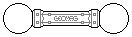Web textodigital.com
The reinforced dodecahedron
The regular dodecahedron is the most unstable and weak of the Platonic Solids, when built with Geomag. Perhaps this is because it encloses the biggest volume, both in absolute terms and relative to the count of its edges: it contains more than 1/4 cubic unit by edge, more than three times the following biggest ratio which is for the cube with 1/12.
Besides this, with three edges meeting at each vertex, it is not possible to balance their magnetism. This is another weakness of this object, although it is shared by the cube and the tetrahedron. As the icosahedron has five edges by vertex, the unbalance is smaller (3:2 instead of 2:1), and the octahedron is the only Platonic Solid with even vertices.
Using pentagonal pyramids instead of panels could seem a good method for strengthening the object, which would also balance all its vertices. Unfortunately, it is not possible to place all the required rods firmly enough, as we explain in this page.
We show now a mixed structure, with panels and auxiliary rods, which proves quite stronger than the normal dodecahedron stabilized with 12 pentagonal panels. Beginning with a trihedral angle made of three pentagons, we join the outer edges of the pentagons with three squares which meet, in turn, in a central triangle (this is similar to the last steps of this reinforced rhombicosidodecahedron):
Two such modules, joined by six rods, will complete the dodecahedron:
If built appropriately, eighteen vertices will be balanced. The only vertices which remain unbalanced as in a normal dodecahedron are those of both trihedral angles, where still three rods and three pentagons meet.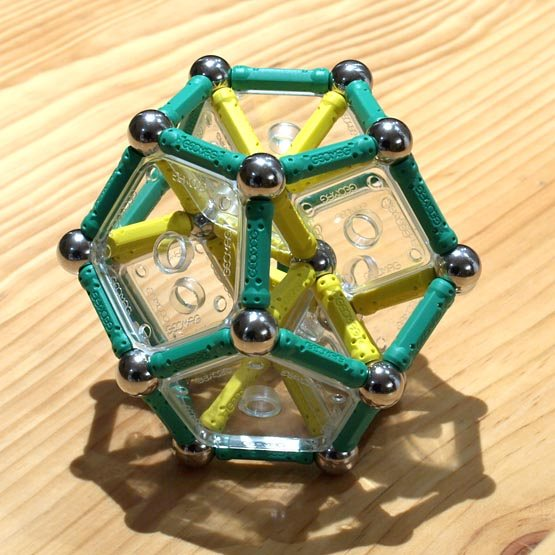The reinforced regular dodecahedron 92 pieces: 26 balls, 54 rods, 6 pentagons, 6 squares (506.32 g)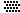If we need, or want all vertices balanced, we could join those two vertices with three rods and two balls (shown in red in the following pictures). Strictly speaking, we could balance them with just two rods, but that would leave their other ends floating around. Ideally, the unbalanced vertices were of opposite polarities.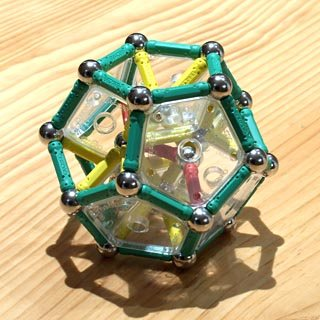The balanced, reinforced regular dodecahedron 97 pieces: 28 balls, 57 rods, 6 pentagons, 6 squares (537.62 g)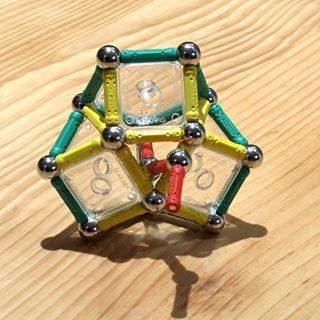I tested the relative strength of both dodecahedra loading them with three quite heavy books. The normal dodecahedron (in the following left-hand picture), supported the intermediate book but couldn't resist when I added the lightest one. I don't think this is bad at all, as that book was quite heavy. However, the reinforced version resisted all three books at once (right-hand picture). It collapsed when I added a fourth book similar to the lightest one.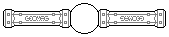Karl Horton made more precise measurements of the strength of the reinforced dodecahedron, as he shows in this video. According to his experiment, the object resists loads up to 6.8 kg. He also made a similar test for the normal dodecahedron, which would resist loads up to 3.0 kg.